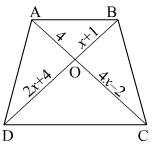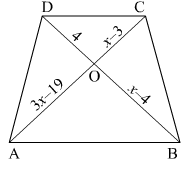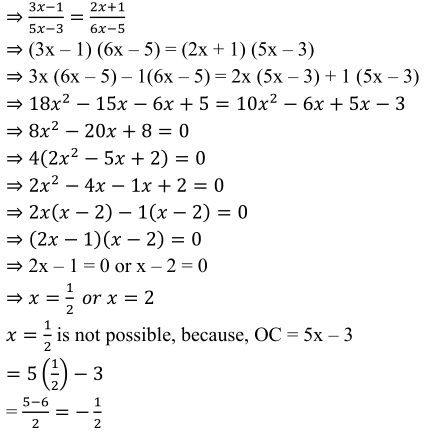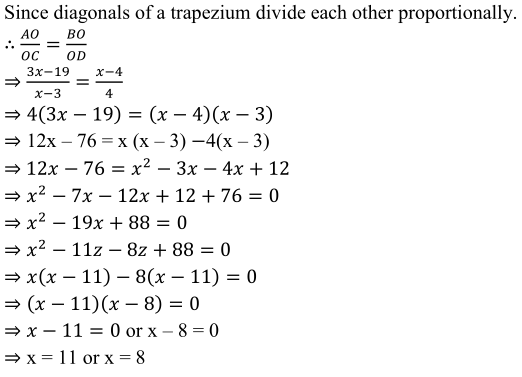## Chapter 4 triangles R.D. Sharma Solutions for Class 10th Math Exercise 4.4

Exercise 4.4

1. (i) In below fig., If AB || CD, find the value of x.(ii) In the below fig., If AB || CD, find the value of x.(iii) In below fig., AB || CD. If OA = 3x – 19, OB = x – 4, OC = x – 3 and OD = 4, find x.Solution

(i)(ii)(iii)